This site uses cookies. By continuing to browse the ConceptDraw site you are agreeing to our Use of Site Cookies.
ConceptDraw Samples | Computer and networks — Rack diagrams

# Rack Diagrams

Samples of rack diagrams are created with ConceptDraw DIAGRAM diagramming and vector drawing software enhanced with Rack Diagrams solution from ConceptDraw Solution Park.

ConceptDraw DIAGRAM provides export of vector graphic multipage documents into multiple file formats: vector graphics (SVG, EMF, EPS), bitmap graphics (PNG, JPEG, GIF, BMP, TIFF), web documents (HTML, PDF), PowerPoint presentations (PPT), Adobe Flash (SWF).

## Tutorials and Solutions:

Video Tutorials: ConceptDraw Solution Park

Solutions: Rack Diagrams for ConceptDraw DIAGRAM

## Sample 1: Application Server Rack Diagram

Rack diagrams sample: Application server rack diagram.

This example is created using ConceptDraw DIAGRAM diagramming software enhanced with Rack Diagrams solution from ConceptDraw Solution Park.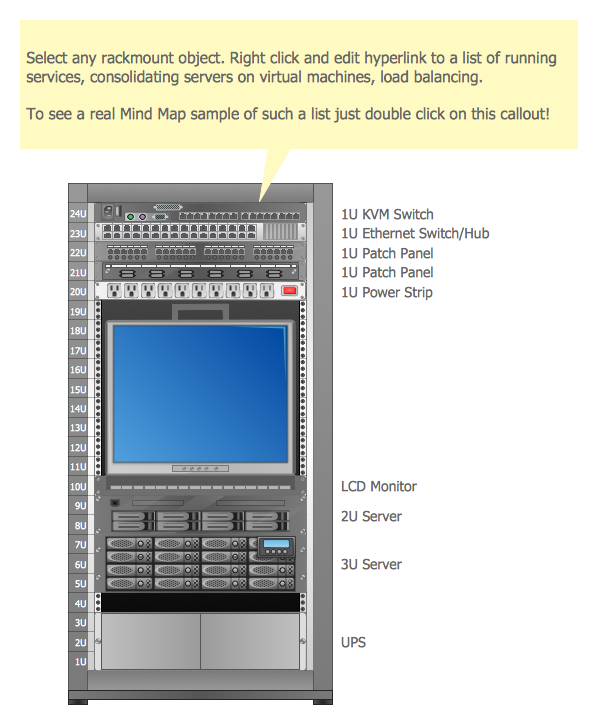## Sample 2: Rack Diagram

Rack diagrams sample: Rack diagram.

This example is created using ConceptDraw DIAGRAM diagramming software enhanced with Rack Diagrams solution from ConceptDraw Solution Park.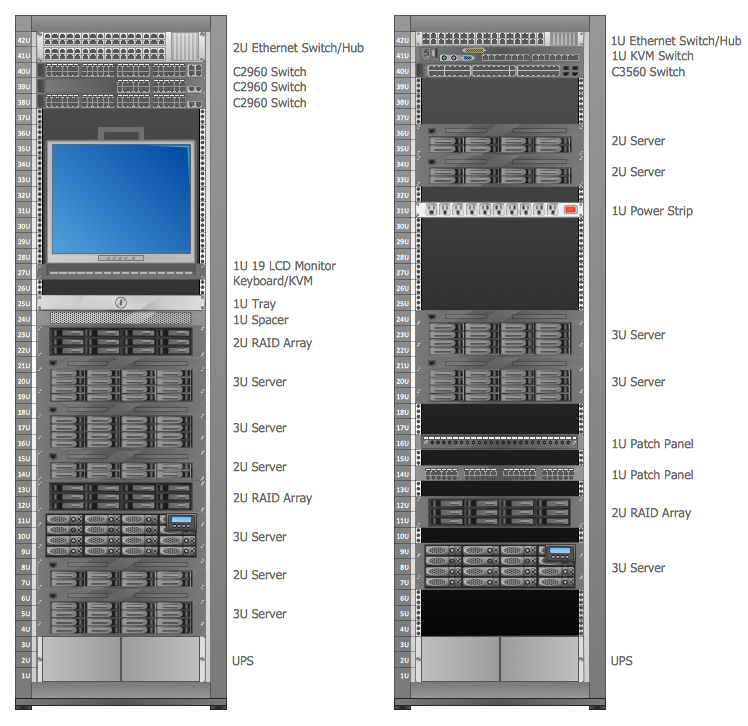## Sample 3: Rack Diagram — Virtualized Computer Center

Rack diagrams sample: Virtualized computer center.

This example is created using ConceptDraw DIAGRAM diagramming software enhanced with Rack Diagrams solution from ConceptDraw Solution Park.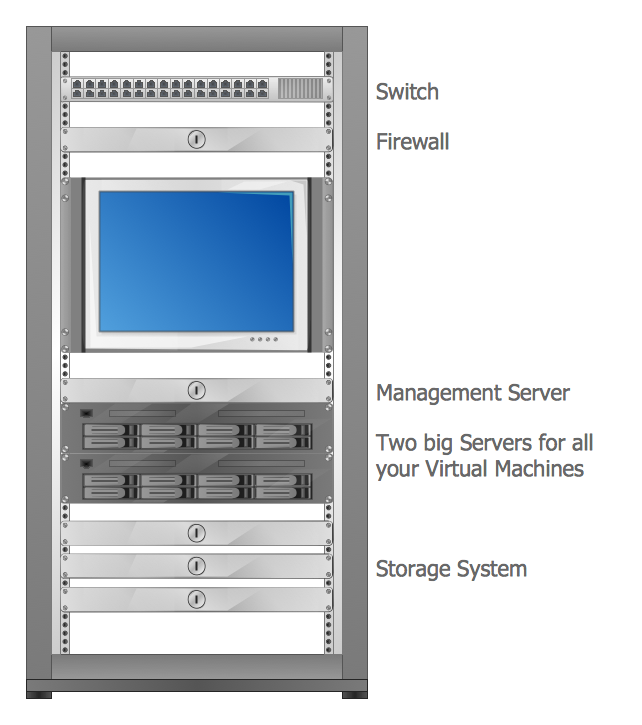## Sample 4: Rack Diagram — Rackunit

Rack diagrams sample: Rackunit.

This example is created using ConceptDraw DIAGRAM diagramming software enhanced with Rack Diagrams solution from ConceptDraw Solution Park.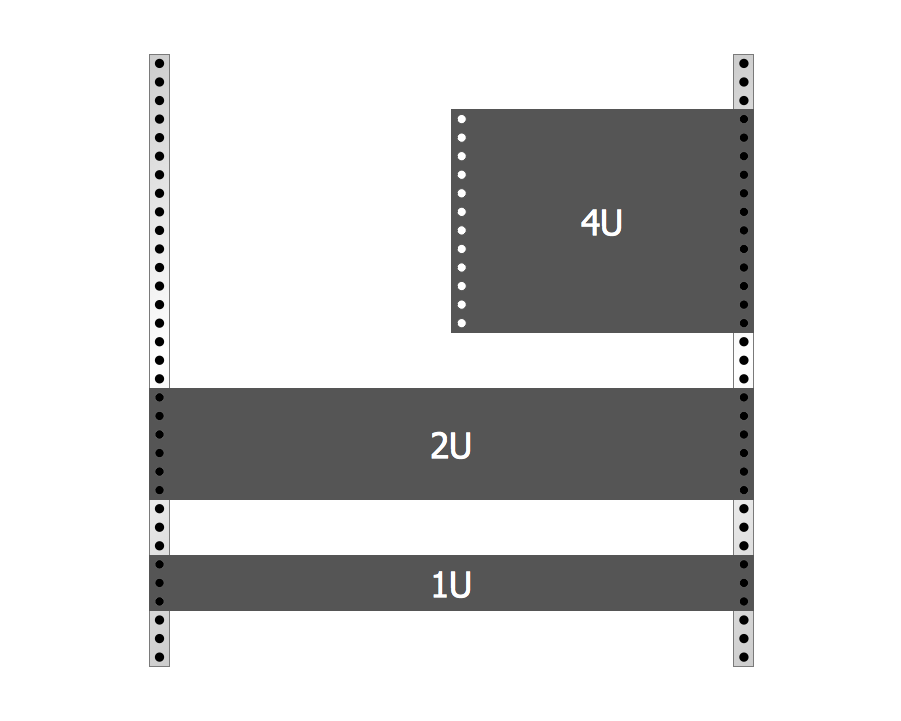## Sample 5: Typical Server Rack Diagram

Rack diagrams sample: Typical server rack diagram.

This example is created using ConceptDraw DIAGRAM diagramming software enhanced with Rack Diagrams solution from ConceptDraw Solution Park.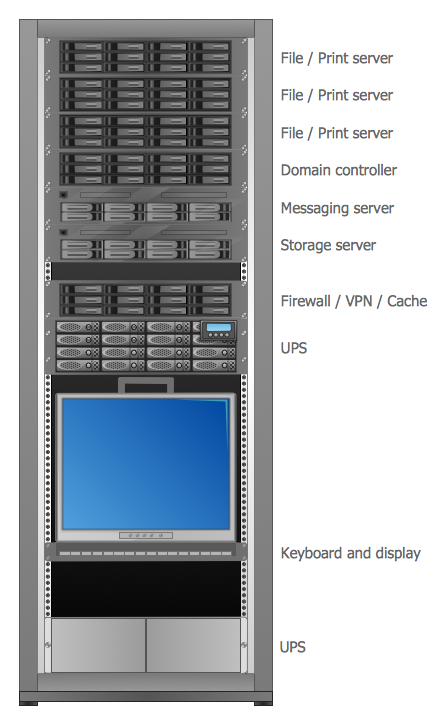## Sample 6: Rack Diagram — Servers

Rack diagrams sample: Servers.

This example is created using ConceptDraw DIAGRAM diagramming software enhanced with Rack Diagrams solution from ConceptDraw Solution Park.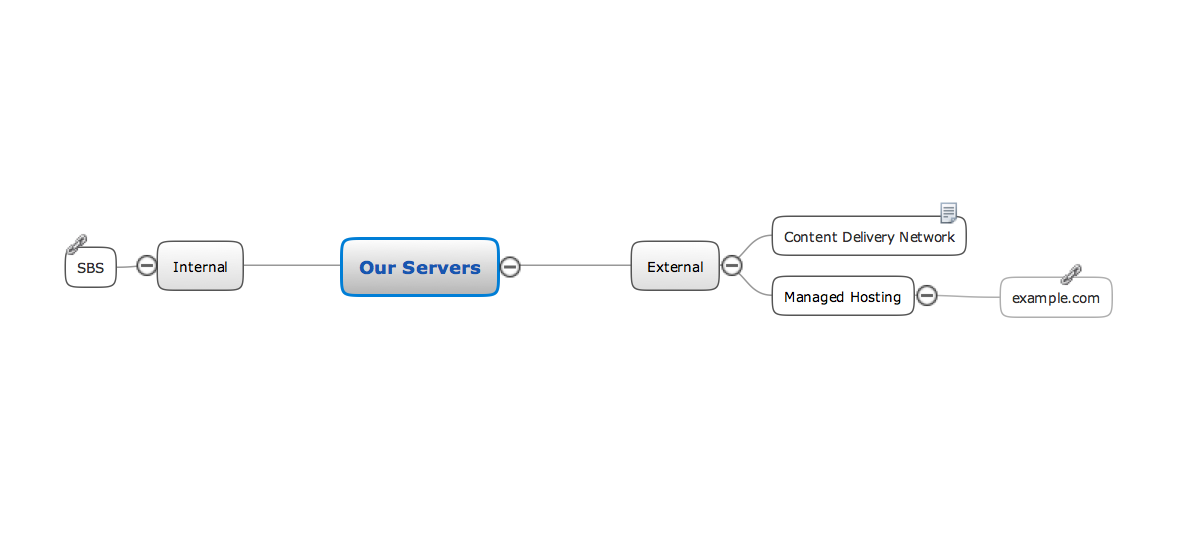All samples are copyrighted CS Odessa's.

Usage of them is covered by Creative Commons “Attribution Non-Commercial No Derivatives” License.

The text you can find at: https://creativecommons.org/licenses/by-nc-nd/3.0